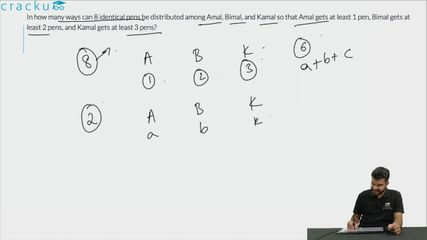Question 95

# In how many ways can 8 identical pens be distributed among Amal, Bimal, and Kamal so that Amal gets at least 1 pen, Bimal gets at least 2 pens, and Kamal gets at least 3 pens?

Solution

After Amal, Bimal and Kamal are given their minimum required pens, the pens left are 8 - (1 + 2 + 3) = 2 pens
Now these two pens have to be divided between three persons so that each person can get zero pens = $$^{2+3-1}C_{3-1}$$ = $$^4C_2$$  = 6

### View Video Solution